Try out our new practice tests completely free!# Quiz 15: Active Filters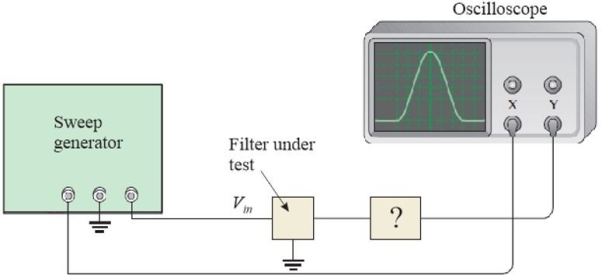Figure 4 A test of a filter using an oscilloscope -Refer to Figure 4. The signal on the X channel of the oscilloscope is a(n)(Multiple Choice)

AThe critical frequency of a high- pass filter is where the response is(Multiple Choice)

CA high Q bandpass filter(Multiple Choice)

B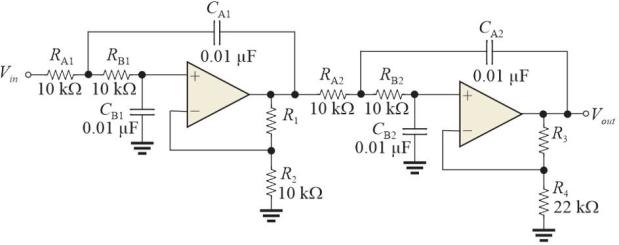Figure 1 Stage 1: DF = 1.848 and R1/R2 = 0.152; stage 2: DF = 0.765 and R3/R4 =1.235. -Refer to Figure 1 with values as given. R3 should have a value of
(Multiple Choice)(Multiple Choice)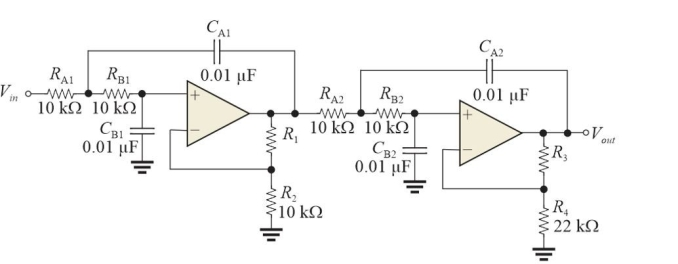Figure 1 Stage 1: DF = 1.848 and R1/R2 = 0.152; stage 2: DF = 0.765 and R3/R4 =1.235. -Refer to Figure 1 with values as given. R1 should have a value of
(Multiple Choice)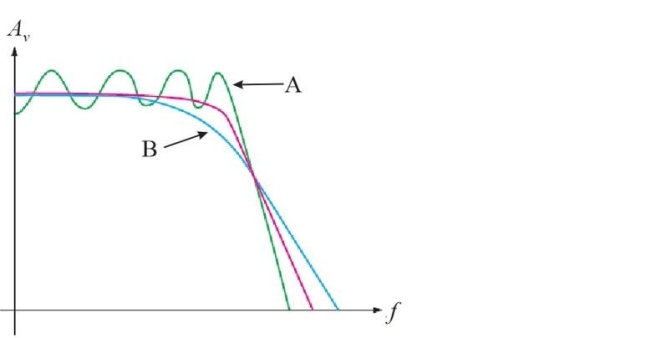Figure 5 Response curve for three different filters -Refer to Figure 5. The response labeled "A" represents a
(Multiple Choice)A low- pass filter has a critical frequency of 10 kHz. The bandpass is
(Multiple Choice)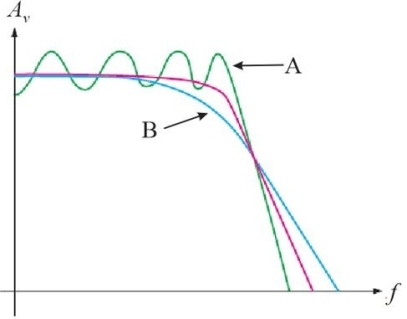Figure 5 Response curve for three different filters -Refer to Figure 5. The response labeled "B" represents a
(Multiple Choice)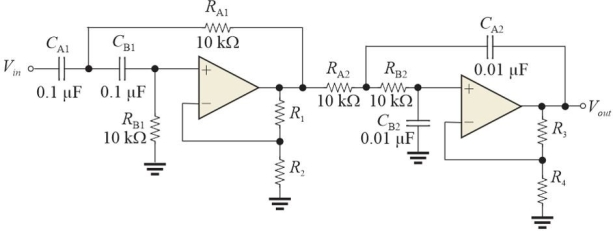Figure 2 Note that the capacitors have different values. -Refer to Figure 2. The fc for the first section is
(Multiple Choice)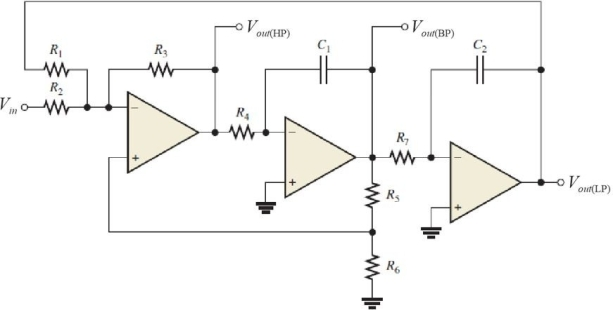Figure 3 -Refer to Figure 3. This type of filter is called a
(Multiple Choice)The bandwidth of a bandpass filter is measured at
(Multiple Choice)The best response to use for pulses is
(Multiple Choice)A basic one- pole RC low- pass filter has fc = 5.9 kHz using a 0.01 µF capacitor. The resistor value is
(Multiple Choice)Figure 1 Stage 1: DF = 1.848 and R1/R2 = 0.152; stage 2: DF = 0.765 and R3/R4 =1.235. -Refer to Figure 1. Assume Vin = 1.0 Vpp sine wave at 20 kHz. At this frequency, Vout is approximately
(Multiple Choice)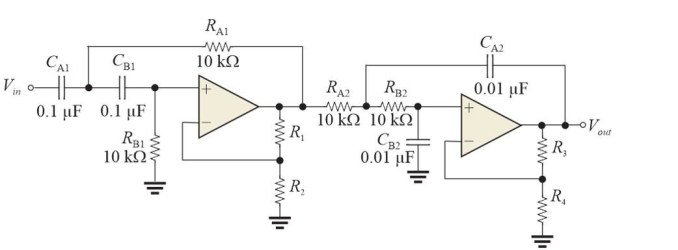Figure 2 Note that the capacitors have different values. -Refer to Figure 2. Assume the capacitors in section 1 are made larger. This change will
(Multiple Choice)Figure 4 A test of a filter using an oscilloscope -Refer to Figure 4. The block with the ? on it represents a
(Multiple Choice)Figure 3 -Refer to Figure 3. An advantage of this type of filter is that it
(Multiple Choice)Assume a high- pass filter and a low- pass filter are cascaded to form a band- pass filter. The upper cutoff frequency will be determined by
(Multiple Choice)Figure 4 A test of a filter using an oscilloscope -Refer to Figure 4. The input signal to the filter (Vin)is a(n)
(Multiple Choice)## Filters

• Essay(0)
• Multiple Choice(0)